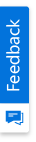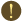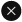We use cookies to give you the best experience on our website. If you continue to browse, then you agree to our privacy policy and cookie policy.Unfortunately, activation email could not send to your email. Please try again.# What are the rendering modes available for pyramid series in the Chart?

Platform: ASP.NET MVC |
Control: Chart
Tags:

 Essential Chart supports rendering of pyramid series in surface and linear mode by using the property PyramidMode of series. Linear mode In Linear mode, the pyramid series is rendered such that the height of a pyramid segment is proportional to the y value of the point represented by that segment. To render pyramid series in Linear mode, you can set the PyramidMode property to Linear. For example, consider a pyramid series having two points with y values [50, 50], then the pyramid series is rendered such that the height of the two segments are proportional to their y values. In this case, the two pyramid segments are drawn such that the height of each segment is 50% of pyramid height. In general, when y values of two points in pyramid series are same, then the height of their pyramid segments are equal in linear mode. Refer to the following code example. CSHTML ```@(Html.EJ().Chart("container")     .Series(ser =>         {             ser.Points(point =>                 {                     point.X("1").Y(50).Add();                     point.X("2").Y(50).Add();                                    })                 .PyramidMode(PyramidMode.Linear)                 .Type(SeriesType.Pyramid).Add();         }) ) ```  The following screenshot displays the pyramid series with two points having the same y values in linear mode. Since both the points have same y values, the height of the two pyramid segments are equal.Surface mode In Surface mode, the pyramid series is rendered such that the surface area of a pyramid segment is proportional to the y value of the point represented by that segment. To render pyramid series in surface mode, you can set the PyramidMode property to Surface. For example, consider a pyramid series having two points in the surface mode with y values [50, 50]. The Pyramid series is rendered such that area of the segment is proportional to the y values. In this case, both the segments consume 50% of the total surface area of the pyramid chart. In general, when y values of the two points in pyramid series are equal, then the area of their pyramid segments are equal in surface mode. Refer to the following code example. CSHTML ```@(Html.EJ().Chart("container")     .Series(ser =>         {             ser.Points(point =>                 {                     point.X("1").Y(50).Add();                     point.X("2").Y(50).Add();                                    })                 .PyramidMode(PyramidMode.Surface)                 .Type(SeriesType.Pyramid).Add();         }) ) ```  The following screenshot displays a pyramid series in surface mode with two points having the same y values.2X faster development

The ultimate ASP.NET MVC UI toolkit to boost your development speed.You are using an outdated version of Internet Explorer that may not display all features of this and other websites. Upgrade to Internet Explorer 8 or newer for a better experience.